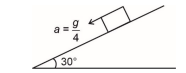# JEE Main 2024 Daily Practice Questions for 23 September 2023

Make a habit of solving JEE Main 2024 Daily Practice Questions on a regular basis. Here the candidates can check out the questions for 23rd September and start to solve them.JEE Main 2024 Daily Practice Questions for 23 September 2023

JEE Main 2024 Daily Practice Questions: Here the candidates will find out the JEE Main 2024 daily practice questions for 23rd September 2023. It is a good habit to solve daily practice questions before the JEE Masin 2024 exam. Since it is one of the most vital entrance tests for pursuing engineering after class 12, therefore, solving the daily practice questions help the candidates to maintain consistency in their performance level.

## JEE Main 2024 Daily Practice Questions

Go through the JEE Main 2024 daily practice questions for 23rd September here:

### Physics:

Ques. A car is moving on a circular track with a of radius 50 cm and a coefficient of friction being 0.34. On this horizontal track, the maximum safe speed for turning is equal to (g= 10m/s2)

1. 1.03

2. 1.7

3. 1.3

4. 1.8

Ques. Find the ratio of the maximum wavelength of the Lyman series of Hydrogen atoms to the minimum wavelength of the Balmer series of helium atoms.

1. 4/3

2. 1

3. 3/2

4. 3/4

Ques. A block is sliding down an inclined plane of inclination 30°, with an acceleration of g/4:Find the coefficient of friction between the block and incline.

1. 1/√3

2. 1/ 2√3

3. 1/3

4. 1/2

### Chemistry:

Ques. Which of the following molecules has the highest bond disassociation energy?

1. I2

2. F2

3. Cl2

4. Br2

Ques. Select the correct statement among the following.

1. Photochemical smog has a high concentration of oxidizing agent

2. Classical smog has a high concentration of oxidizing agent

3. Classical smog contains NO2

4. None of these

Ques. Which of the following compound(s) is/are paramagnetic?

1. NO2

2. NO

3. K2O

4. Na2O2

### Mathematics:

Ques. Let P (h,k) be two points on x2= 4y which is at the shortest distance from Q (0, 33) then the difference of distances of P (h, k) from directrix of y2= 4 (x+y) is:

1. 2

2. 4

3. 6

4. 8

Ques. Consider the data: x, y, 10, 12, 4, 6, 8, 12. If the mean is 9 and the variance is 9.25, then the value of 3x-2y is (x>y)

1. 25

2. 1

3. 24

4. 13

Ques. If the coefficients of three consecutive terms in the expansion of (1+x)n are in the ratio 1:5:20; then the coefficient of the fourth term of the expansion is:

1. 3654

2. 3658

3. 3600

4. 1000

Stay tuned to CollegeDekho for more Education News pertaining to entrance exams and admission. You can also write to us at our E-Mail ID news@collegedekho.com.

/news/jee-main-2024-daily-practice-questions-for-23-september-2023-45410/

### Do you have a question? Ask us.

• Typical response between 24-48 hours

• Get personalized response

• Free of Cost